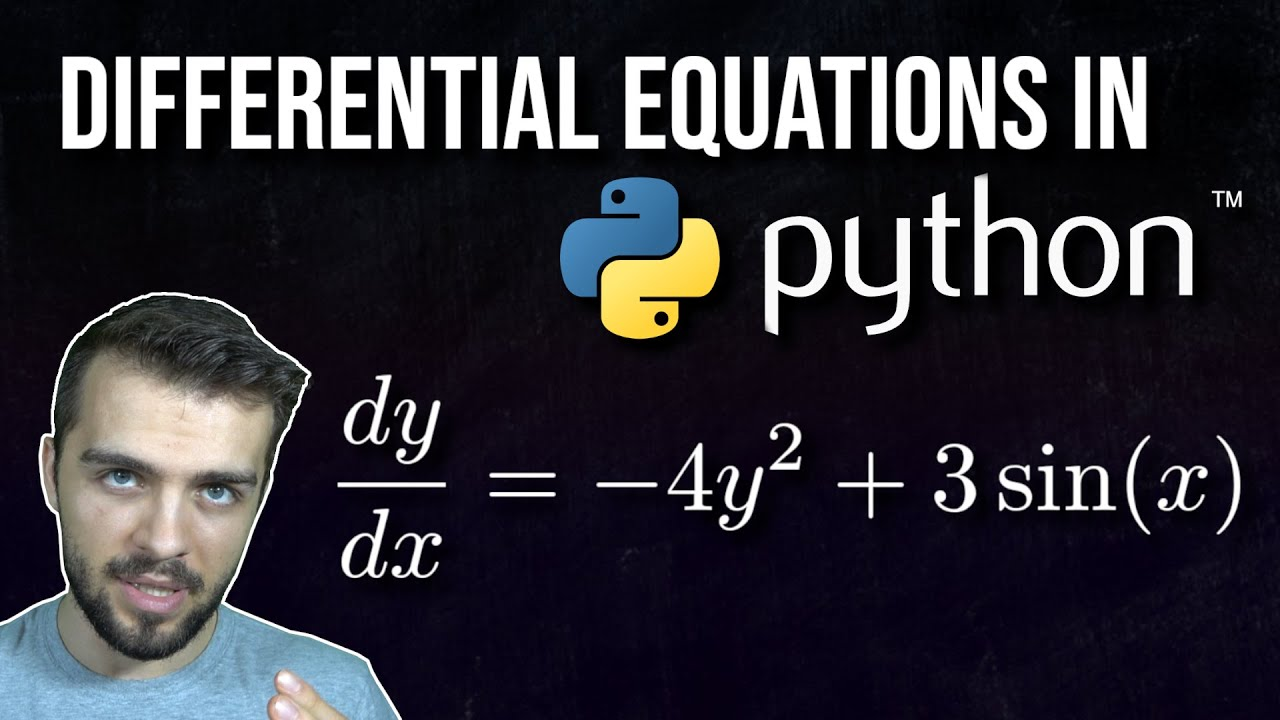# How to Solve Differential Equations in Python (Coupled+Higher Order)Examined are first order ordinary differential equations (ODEs), coupled first order ODEs, and higher order ODEs.

Examined are first order ordinary differential equations (ODEs), coupled first order ODEs, and higher order ODEs.

All code can be found on Github: